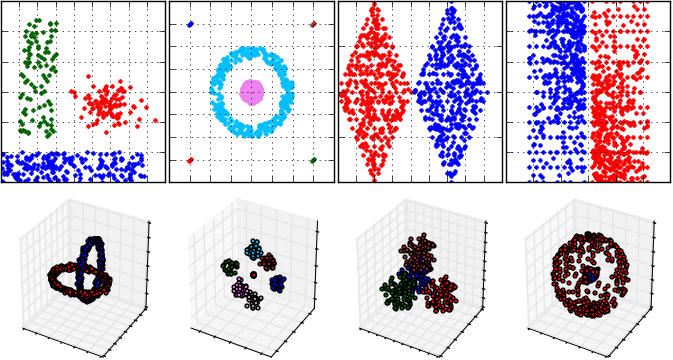PyClustering library

Introduction

PyClustering library is a collection of cluster analysis, graph coloring, travelling salesman problem algorithms, oscillatory and neural network models, containers, tools for visualization and result analysis, etc. High performance is ensured by CCORE library that is a part of the pyclustering library where almost the same algorithms, models, tools are implemented. There is ability to use python code implementation only or CCORE (C/C++) implementation using special flag. CCORE library does not use python.h interface to communicate with python code due to requirement to save ability to use CCORE library or C/C++ code part of CCORE in other projects.

PyClustering consists of six general modules where the algorithms, models, tools are placed:

Cluster analysis algorithms and methods (module pyclustering.cluster):

Oscillatory and neural network models (module pyclustering.nnet):

Graph coloring algorithms (module pyclustering.gcolor):

Containers (module pyclustering.container):

Utils that can be used for analysis, visualization, etc are placed in module pyclustering.utils.

Installation

The simplest way to install pyclustering library is to use pip:

pip3 install pyclustering

The library can be compiled and manually installed on linux machine wherever you want:

# extract content of the pyclustering library...
# compile CCORE library (core of the pyclustering library).
cd pyclustering/ccore
make ccore
cd ../
# add current folder to python path
PYTHONPATH=`pwd`
export PYTHONPATH=\${PYTHONPATH}

The library CCORE for 64-bit windows is distributed with pyclustering library so there is no need to re-built it. If you want to re-built CCORE library you can open CCORE Microsoft Visual Studio project that is located in ccore/ folder and compile it.

Examples

The library provides intuitive and friendly interface, cluster analysis can be easily performed:

# an example of clustering by BIRCH algorithm.
from pyclustering.cluster.birch import birch;
# load data from the FCPS set that is provided by the library.
# create BIRCH algorithm for allocation three objects.
birch_instance = birch(sample, 3);
# start processing - cluster analysis of the input data.
birch_instance.process();
# allocate clusters.
clusters = birch_instance.get_clusters();
# visualize obtained clusters.
visualizer = cluster_visualizer();
visualizer.append_clusters(clusters, sample);
visualizer.show();

Clustering algorithms can be used for image processing:

# an example of image color segmentation.
from pyclustering.samples.definitions import IMAGE_SIMPLE_SAMPLES;
from pyclustering.cluster.kmeans import kmeans;
# load image from the pyclustering collection.
# set initial centers for K-Means algorithm.
start_centers = [ [153, 217, 234, 128], [0, 162, 232, 128], [34, 177, 76, 128], [255, 242, 0, 128] ];
# create K-Means algorithm instance.
kmeans_instance = kmeans(data, start_centers);
# start processing.
kmeans_instance.process();
# obtain clusters that are considered as segments.
segments = kmeans_instance.get_clusters();
# show image segmentation results.

An example cluster analysis (that is performed by DBSCAN algorithm) for FCPS samples and visualization of results:Simulation of oscillatory network based on Hodgkin-Huxley neuron model where six synchronous ensembles of oscillators are formed. It means that three features from input data are allocated where each feature is encoded by only one ensemble.

# an example of simulation of oscillatory network based on Hodgkin-Huxley model
from pyclustering.utils import draw_dynamics;
from pyclustering.nnet.hhn import hhn_network, hhn_parameters;
# set period of 400 time units when high strength value of synaptic connection exists from CN2 to PN.
params = hhn_parameters();
params.deltah = 400;
# prepare external stimulus that encode three different features.
stimulus = [0, 0, 25, 25, 47, 47];
# create oscillatory network that has six oscillators.
net = hhn_network(len(stimulus), stimulus, params);
# perform simulation during 1200 steps in 600 time units.
(t, dyn) = net.simulate(1200, 600);
# visualize results of simulation (output dynamic of the network).
draw_dynamics(t, dyn, x_title = "Time", y_title = "V", separate = True);